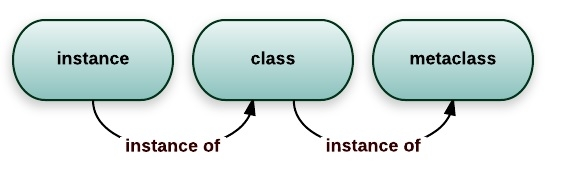# Meta programming with Metaclasses in Python

The term metaprogramming refers to computer programming where the computer programs try to manipulate or have knowledge of itself. Python supports metaprogramming for classes through a new type of class called metaclass.

Meta-programming through metaclasses in python is to build functions and classes which manipulate code by modifying, wrapping or generating existing code.

Key features of meta-programming are −

• Metaclasses
• Decorators
• Class-decorators

## What is a Metaclass

A very confined definition of metaclass can be, a class that creates a class. Python is an object-oriented language where everything is an object and classes are no exception. Defining a class with class keyword, python actually executes it and then generates an object. Since it is an object, you can assign, copy, add attributes, as function parameters, and so on. Using metaclasses allows us to control the generation of classes, such as modifying the properties of a class, checking the legitimacy of the property, and so on.

## Python built-in Metaclass

The metaclass of all classes is the type

class a:
pass

print(type(a))

Output:

<class 'type'>

From the above output, we can see that “a”(class) is an object of class type. We can also say that “a”(class) is an instance of the class type. So type is a metaclass. So in python, every class belongs to built-in metaclass type.## Using Metaclass to create a python class

As we saw in the above example, the type is the default metaclass. We can create a new class with the help of this default metaclass(type).

>>> class hello(object):
pass

can be created manually this way too −

>>> hello = type('hello', (), {})
>>> print(hello)# returns a class object

>>> print(hello()) # creates an instance with the class
<__main__.hello object at 0x05D86830>

Type accepts a dictionary to define the attributes of the class, so

>>> class hello(object):
world = True

Above code is similar to,

hello = type('hello', (), {'world' : True})

## Create a metaclass

So when to create a metaclass? When I want to control the creation of a class, such as verifying or modifying the properties of a class.

The instantiation process of the class −

• Create an instance by calling __new__()
• Call __init__() to initialize the instance created above

So when we creating a custom metaclass, we actually change the parent class’s __new__() or __init__ method.

## Example1 − Metaclass that modified the properties of the class

class LowercaseMeta(type):
def __new__(mcs, name, bases, attrs):
lower_attrs = {}
for k, v in attrs.items():
if not k.startswith('__'):
lower_attrs[k.lower()] = v
else:
lower_attrs[k] = v
return type.__new__(mcs, name, bases, lower_attrs)
class LowercaseClass(metaclass=LowercaseMeta):
BAR = True
def HELLO(self):
print('hello')

print(dir(LowercaseClass))
LowercaseClass().hello()

## Output

['__class__', '__delattr__', '__dict__', '__dir__', '__doc__', '__eq__', '__format__', '__ge__', '__getattribute__', '__gt__', '__hash__', '__init__', '__init_subclass__', '__le__', '__lt__', '__module__', '__ne__', '__new__', '__reduce__', '__reduce_ex__', '__repr__', '__setattr__', '__sizeof__', '__str__', '__subclasshook__', '__weakref__', 'bar', 'hello']
hello

class ListMeta(type):
def __new__(mcs, name, bases, attrs):
attrs['add'] = lambda self, value: self.append(value)
return type.__new__(mcs, name, bases, attrs)
class MyList(list, metaclass=ListMeta):
pass
l = MyList()
print(l)
def class_decorator(cls):
cls.add = lambda self, value: self.append(value)
return cls

@class_decorator
class MyList(list):
pass
l = MyList()
l.append(1)
print(l)

## Output




## The __metaclass__ attribute

In python, either we have a class or one of its bases has a __metaclass__ attributed, it’s taken as the metaclass else type is the metaclass. We can have the __metaclass__ attribute when we write a class as −

So what happens when we define, a class like −

class hello(object):
x = 10

In above, hello class has no __metaclass__ attribute, so the type is used instead, and the class creation is done as −

hello = type(name, bases, dict)

In case our hello does have a metaclass defined −

class hello(object):
__metaclass__ = myMetaClass
pass

Now in the above example, the class creation is done using myMetaClass instead of type. Hello = myMetaClass(name, bases, dict)